# Surfaces and Topology Raymond Flood Gresham Professor of

• Slides: 48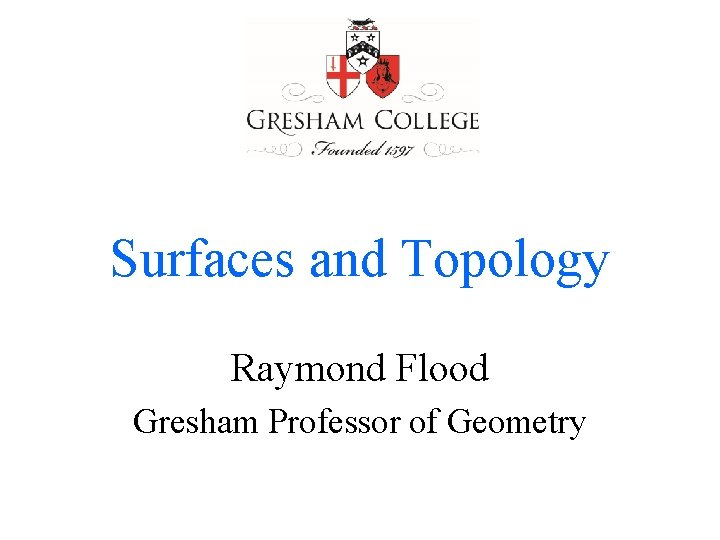Surfaces and Topology Raymond Flood Gresham Professor of GeometryOverview • Leonhard Euler • Difference between geometry and topology - bridges of Königsberg • Euler’s formula for polyhedra – examples, an application and outline of a proof • Classification of surfaces • Hairy ball theoremLeonhard Euler, 1707– 1783 Read Euler, read Euler, he is the master of us all • Notation e for the exponential number, f for a function and i for √− 1. • Infinite series – Euler’s constant (1 + 1/2 + 1/3 + 1/4 + 1/5 +. . . + 1/n) – loge n – Basel problem 1 + 1/4 + 1/9 + 1/16 + 1/25 +. . . = π2/6 4 th powers π4/90 6 th powers π6/945, and up to the 26 th powers! Portrait by Jakob Emanuel Handmann, 1756Euler’s booksThe Exponential Function • Exponential functions 2 n or 3 n or en, where e = 2. 6182818… • ex = 1 + x/1! + x 2/2! + x 3/3! +. . . e = 1 + 1/1! + 1/2! + 1/3! + … • eix = cos x + i sin x • eiπ = − 1 eiπ + 1 = 0 The exponential function ex The slope of this curve at any point x is also exBridges of Königsberg Euler’s drawing of the seven bridges The seven bridges of Königsberg The graph associated with the seven bridges of Königsberg problem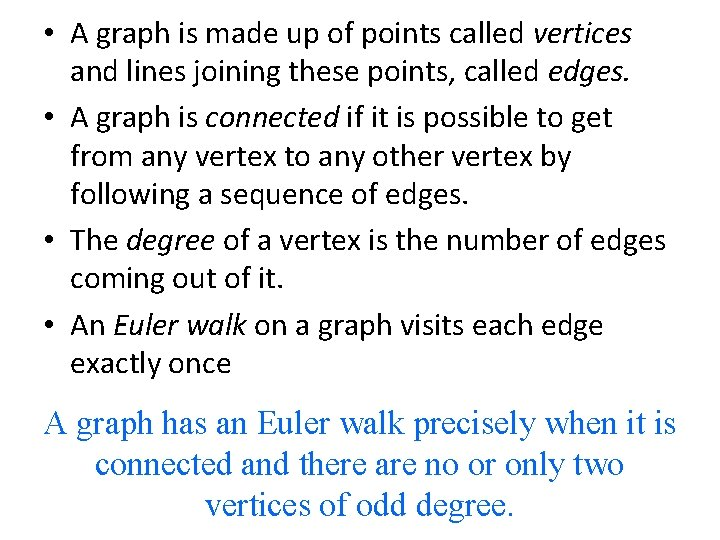• A graph is made up of points called vertices and lines joining these points, called edges. • A graph is connected if it is possible to get from any vertex to any other vertex by following a sequence of edges. • The degree of a vertex is the number of edges coming out of it. • An Euler walk on a graph visits each edge exactly once A graph has an Euler walk precisely when it is connected and there are no or only two vertices of odd degree.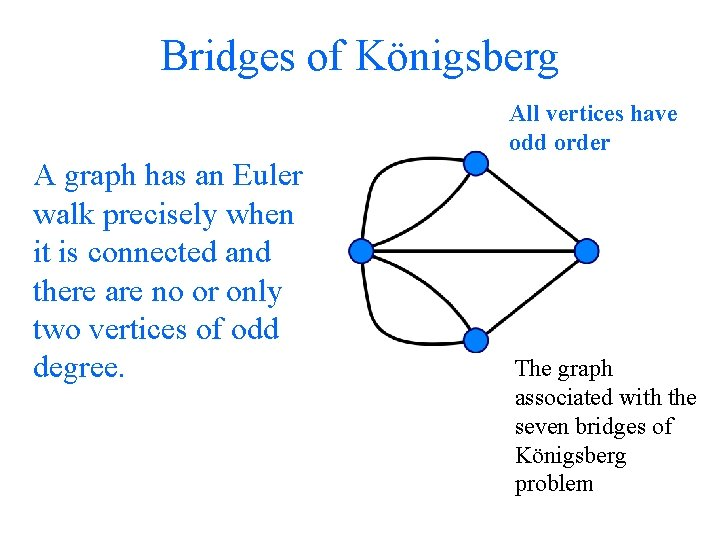Bridges of Königsberg All vertices have odd order A graph has an Euler walk precisely when it is connected and there are no or only two vertices of odd degree. The graph associated with the seven bridges of Königsberg problem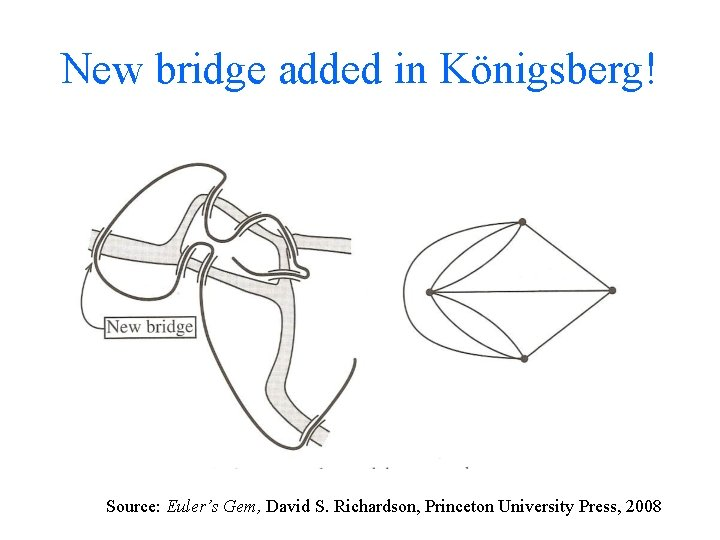New bridge added in Königsberg! Source: Euler’s Gem, David S. Richardson, Princeton University Press, 2008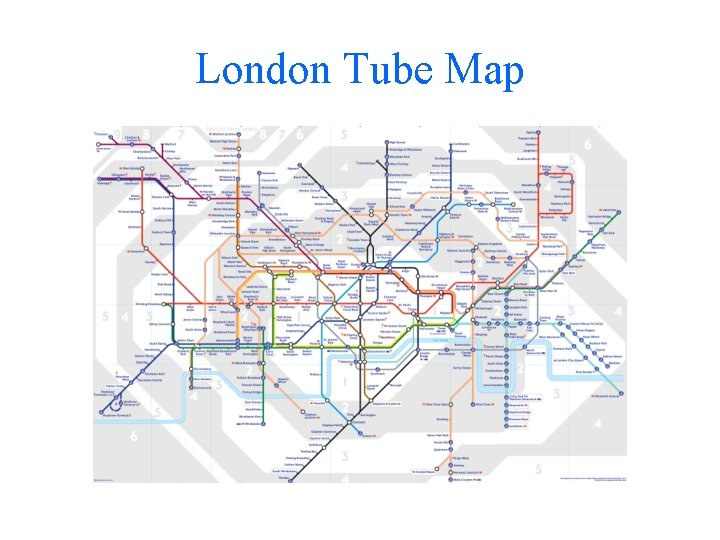London Tube MapGeometry and Topology Geometry • Geometry from the Greek geo- "earth", -metron "measurement" • Concerned with the properties of space and of figures in space. • Concerned with measurement, for example of angles and distances Topology • Topology from the Greek τόπος, "place", and λόγος, "study“ and was originally called analysis situs analysis of position • Concerned with those properties of geometrical figures that are unchanged under continuous deformation – rubber sheet geometry • Topology tackles the question: What shape is thing?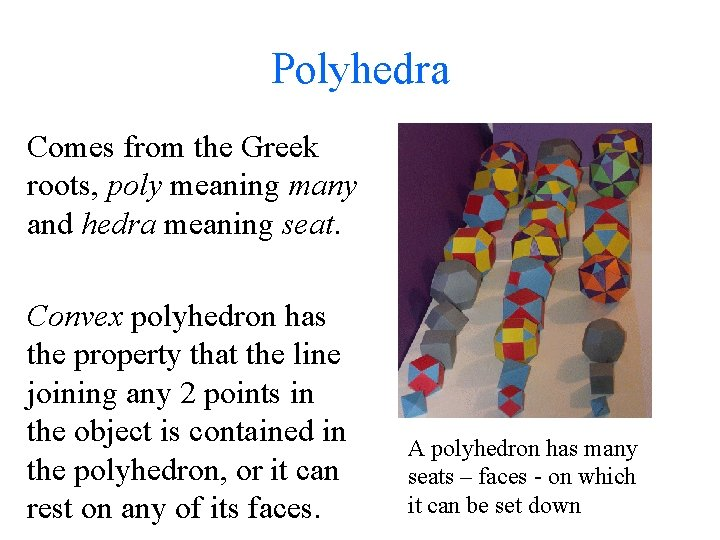Polyhedra Comes from the Greek roots, poly meaning many and hedra meaning seat. Convex polyhedron has the property that the line joining any 2 points in the object is contained in the polyhedron, or it can rest on any of its faces. A polyhedron has many seats – faces - on which it can be set down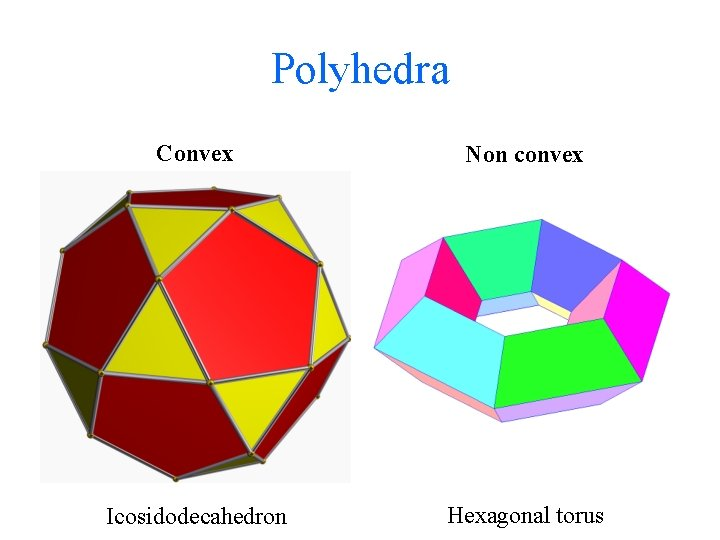Polyhedra Convex Non convex Icosidodecahedron Hexagonal torusEuler’s formula for convex polyhedra, V–E+F=2 V = number of vertices E = number of edges F = number of facesTetrahedron V–E+F=2 Vertices, V = 4 Edges, E = 6 Faces, F = 4 V–E+F=4– 6+4=2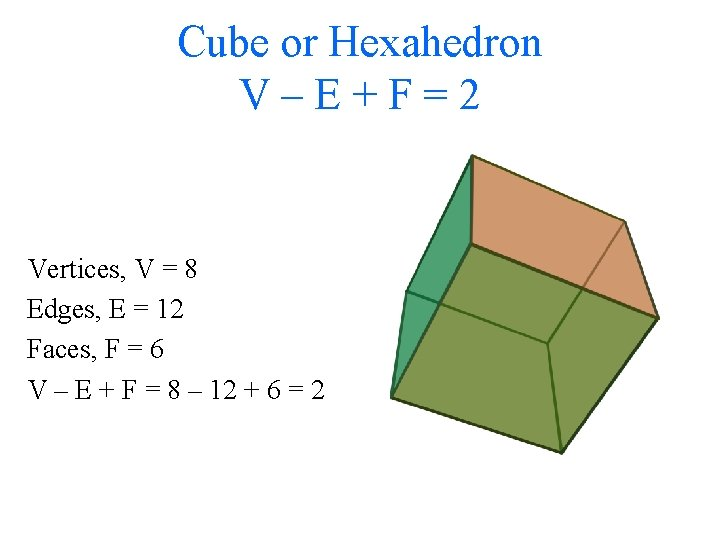Cube or Hexahedron V–E+F=2 Vertices, V = 8 Edges, E = 12 Faces, F = 6 V – E + F = 8 – 12 + 6 = 2Octahedron V–E+F=2 Vertices, V = 6 Edges, E = 12 Faces, F = 8 V – E + F = 6 – 12 + 8 = 2Dodecahedron V–E+F=2 Vertices, V = 20 Edges, E = 30 Faces, F = 12 V – E + F = 20 – 30 + 12 = 2Icosahedron V–E+F=2 Vertices, V = 12 Edges, E = 30 Faces, F = 20 V – E + F = 20 – 30 + 12 = 2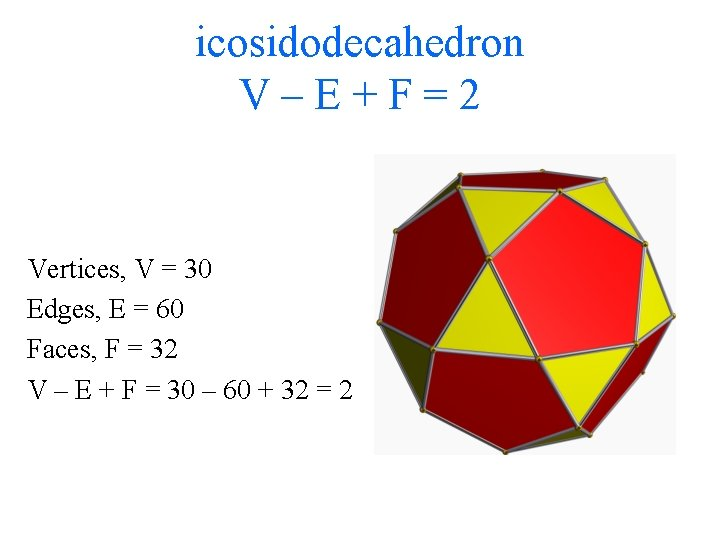icosidodecahedron V–E+F=2 Vertices, V = 30 Edges, E = 60 Faces, F = 32 V – E + F = 30 – 60 + 32 = 2V–E+F • If we remove an edge and a face at the same time then number of vertices – number of edges + number of faces stays the same. Because you are talking away one less edge but adding on one less face • Similarly we remove an edge and a vertex at the same time then number of vertices – number of edges + number of faces stays the same. Because you are talking away one less edge but adding on one less vertex.1: Deform the convex polyhedron into a sphere V – E + F left unchanged2: Remove an edge so as to merge two faces. Leaves V – E + F unchanged Remove this edge, so merging the two adjacent faces into one3: End up with only 1 face and the edges and vertices forming a graph with no loops – a tree Remove this edge, so merging the two adjacent faces into one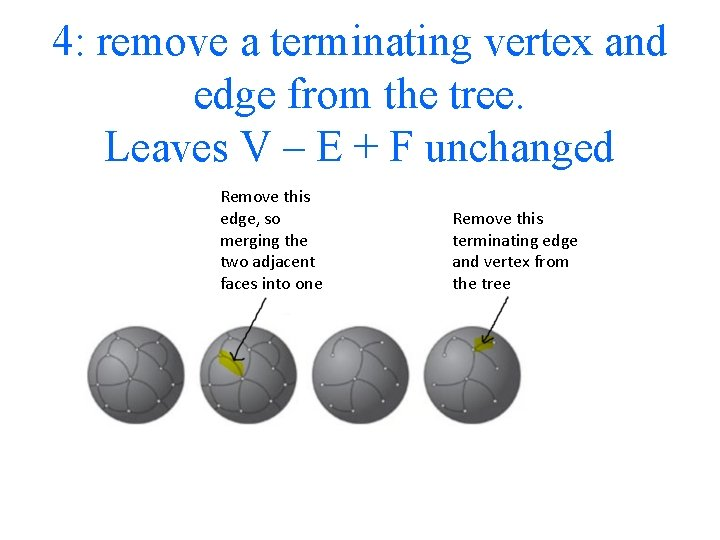4: remove a terminating vertex and edge from the tree. Leaves V – E + F unchanged Remove this edge, so merging the two adjacent faces into one Remove this terminating edge and vertex from the tree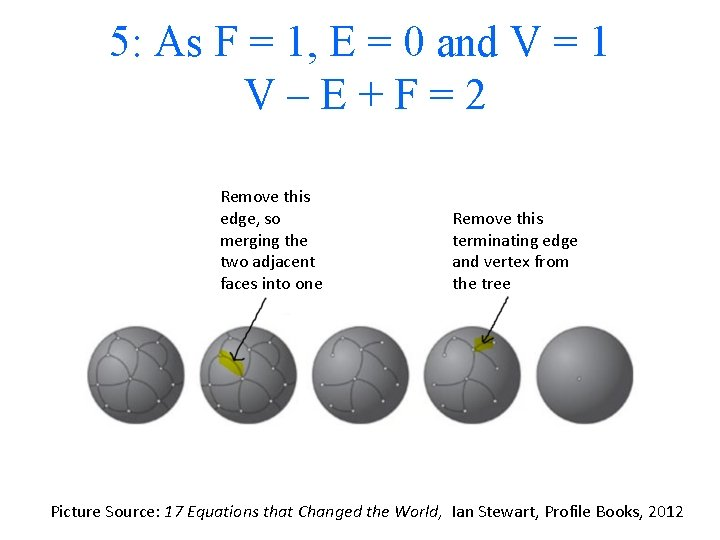5: As F = 1, E = 0 and V = 1 V–E+F=2 Remove this edge, so merging the two adjacent faces into one Remove this terminating edge and vertex from the tree Picture Source: 17 Equations that Changed the World, Ian Stewart, Profile Books, 2012In a regular polyhedron all the faces are the same and the arrangement of faces at each vertex is the same. Tetrahedron – four faces each an equilateral triangle Cube – six faces, each a square Octahedron – eight faces each an equilateral triangle Dodecahedron – twelve faces each a regular pentagon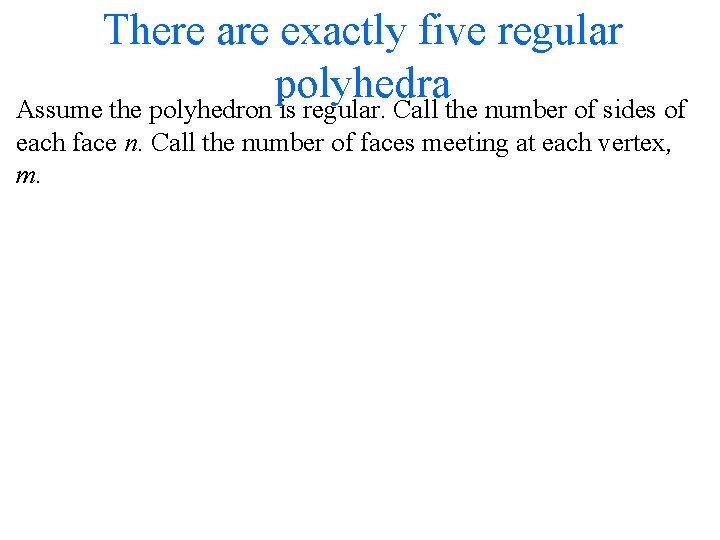There are exactly five regular polyhedra Assume the polyhedron is regular. Call the number of sides of each face n. Call the number of faces meeting at each vertex, m.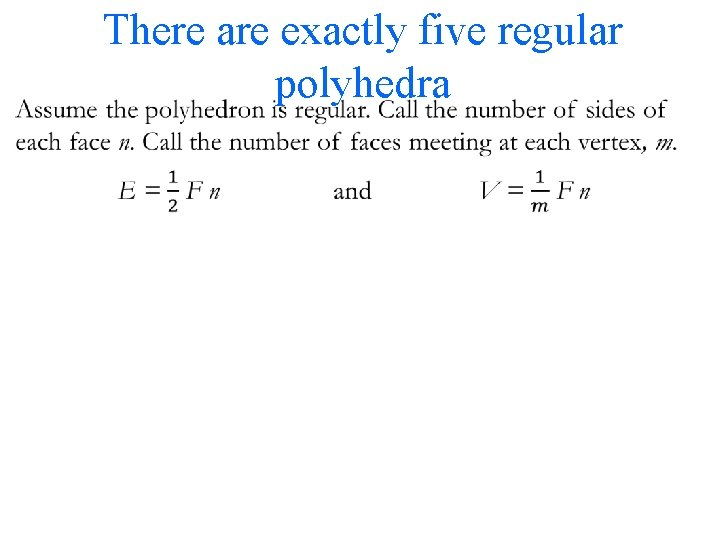• There are exactly five regular polyhedra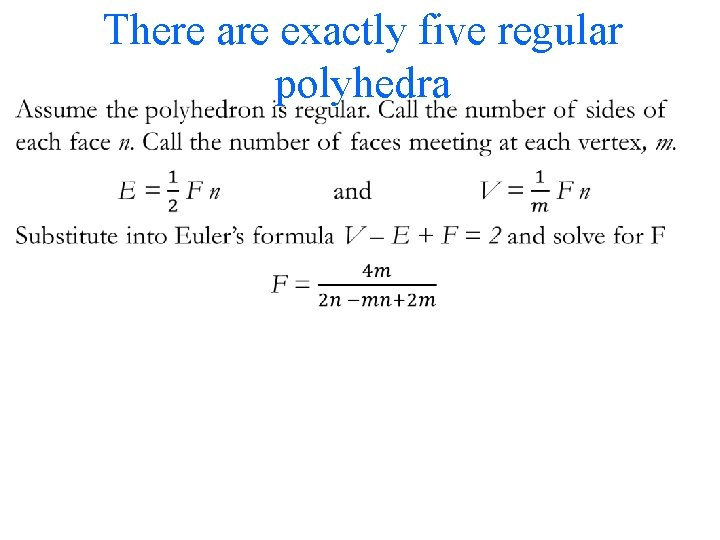• There are exactly five regular polyhedra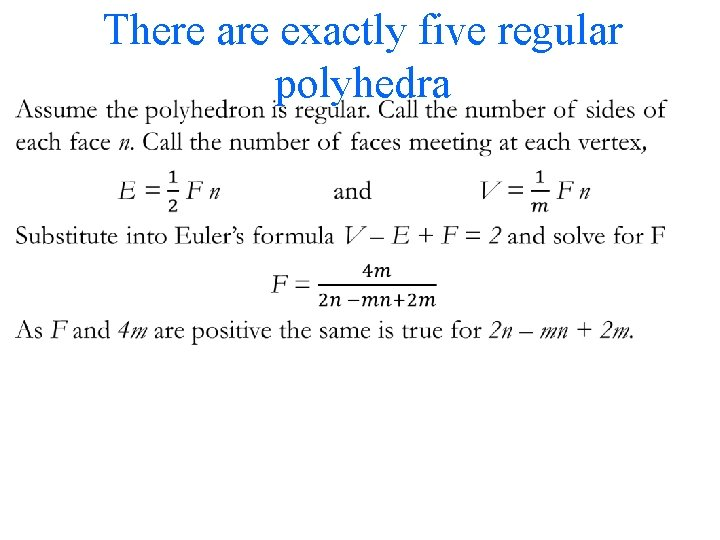• There are exactly five regular polyhedra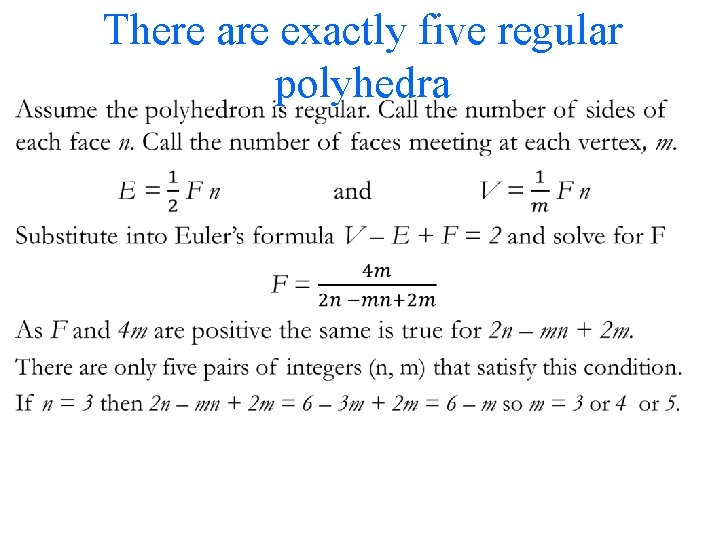• There are exactly five regular polyhedra• There are exactly five regular polyhedra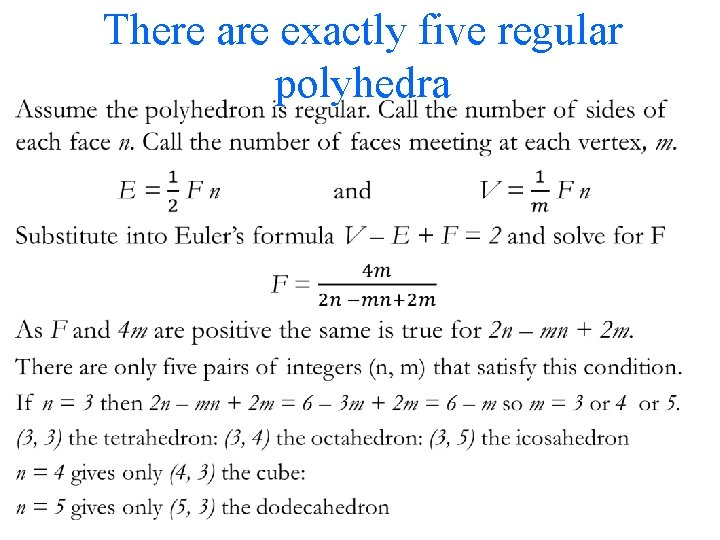• There are exactly five regular polyhedra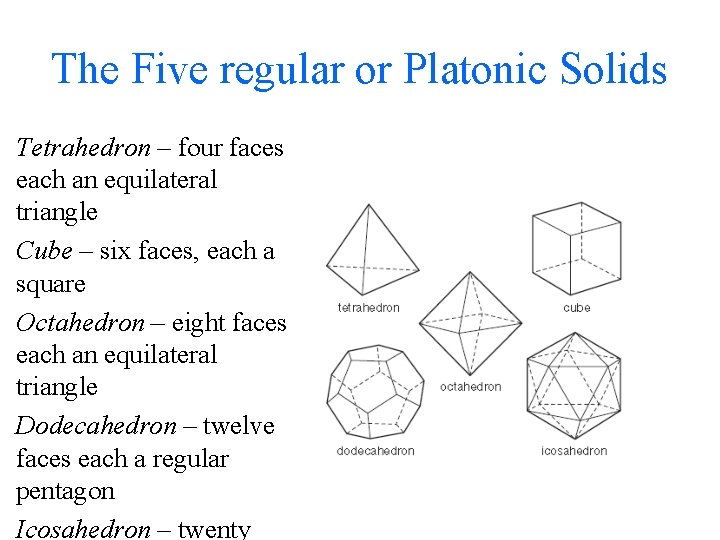The Five regular or Platonic Solids Tetrahedron – four faces each an equilateral triangle Cube – six faces, each a square Octahedron – eight faces each an equilateral triangle Dodecahedron – twelve faces each a regular pentagon Icosahedron – twenty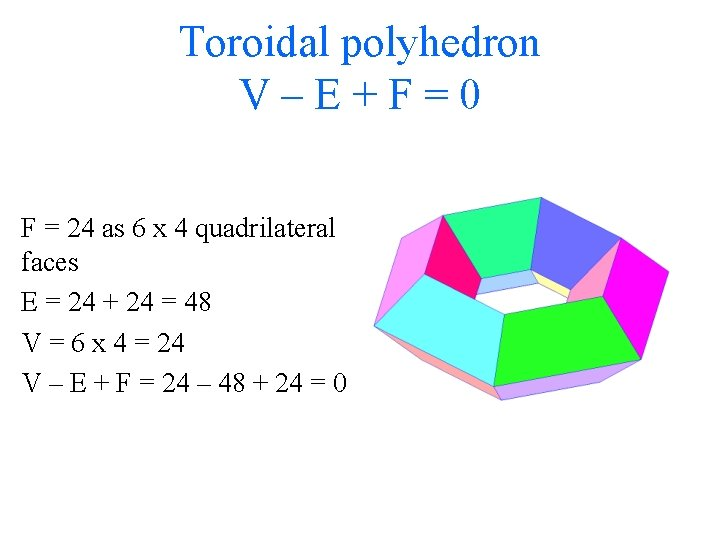Toroidal polyhedron V–E+F=0 F = 24 as 6 x 4 quadrilateral faces E = 24 + 24 = 48 V = 6 x 4 = 24 V – E + F = 24 – 48 + 24 = 0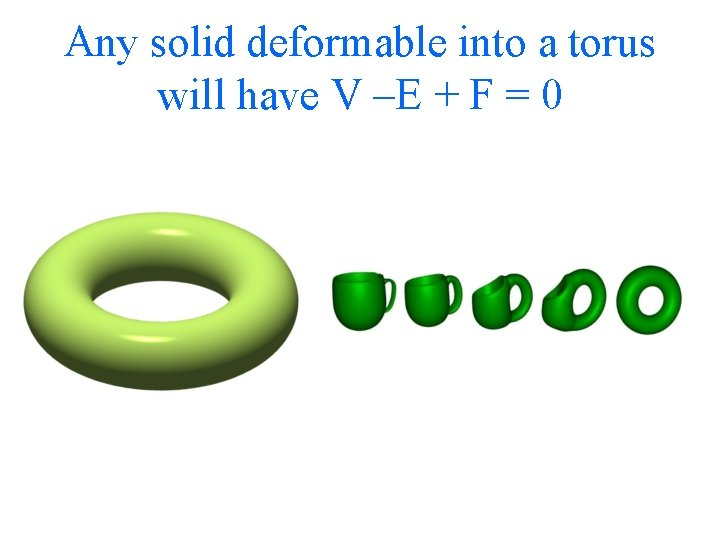Any solid deformable into a torus will have V –E + F = 0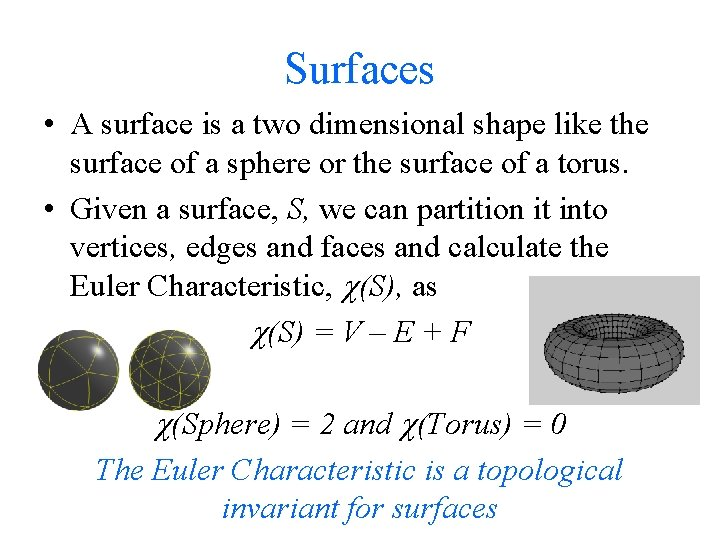Surfaces • A surface is a two dimensional shape like the surface of a sphere or the surface of a torus. • Given a surface, S, we can partition it into vertices, edges and faces and calculate the Euler Characteristic, (S), as (S) = V – E + F (Sphere) = 2 and (Torus) = 0 The Euler Characteristic is a topological invariant for surfaces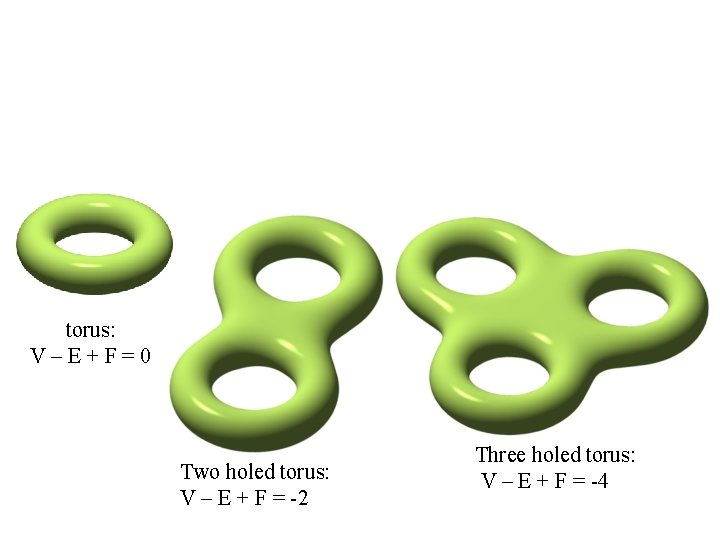torus: V–E+F=0 Two holed torus: V – E + F = -2 Three holed torus: V – E + F = -4Any solid deformable into a g holed torus satisfies V – E + F = 2 – 2 g torus: V–E+F=0 Two holed torus: V – E + F = -2 Three holed torus: V – E + F = -4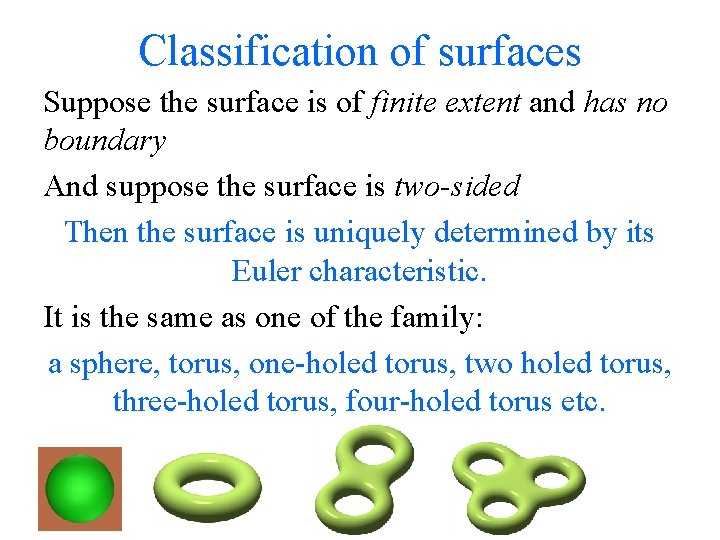Classification of surfaces Suppose the surface is of finite extent and has no boundary And suppose the surface is two-sided Then the surface is uniquely determined by its Euler characteristic. It is the same as one of the family: a sphere, torus, one-holed torus, two holed torus, three-holed torus, four-holed torus etc.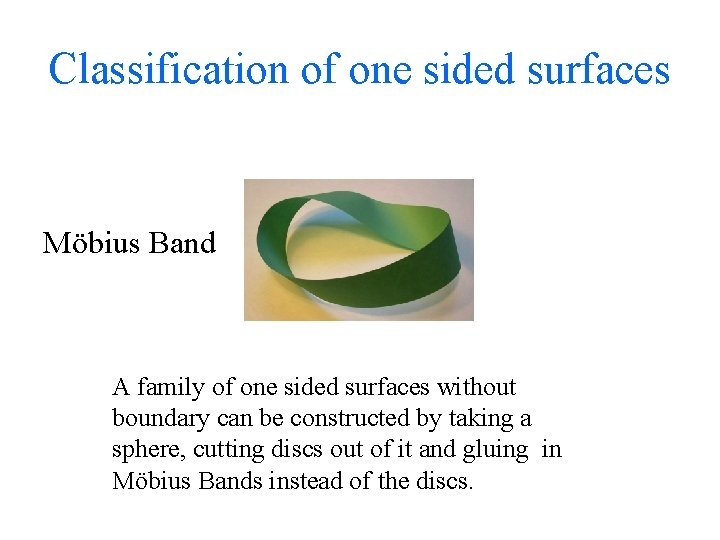Classification of one sided surfaces Möbius Band A family of one sided surfaces without boundary can be constructed by taking a sphere, cutting discs out of it and gluing in Möbius Bands instead of the discs.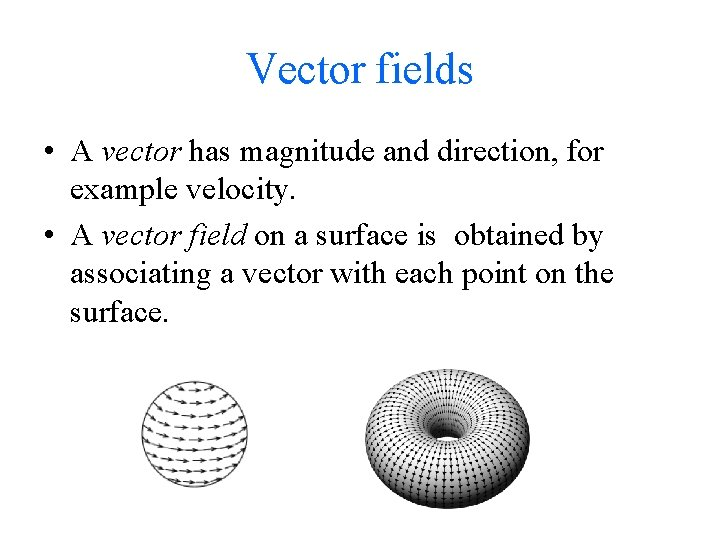Vector fields • A vector has magnitude and direction, for example velocity. • A vector field on a surface is obtained by associating a vector with each point on the surface.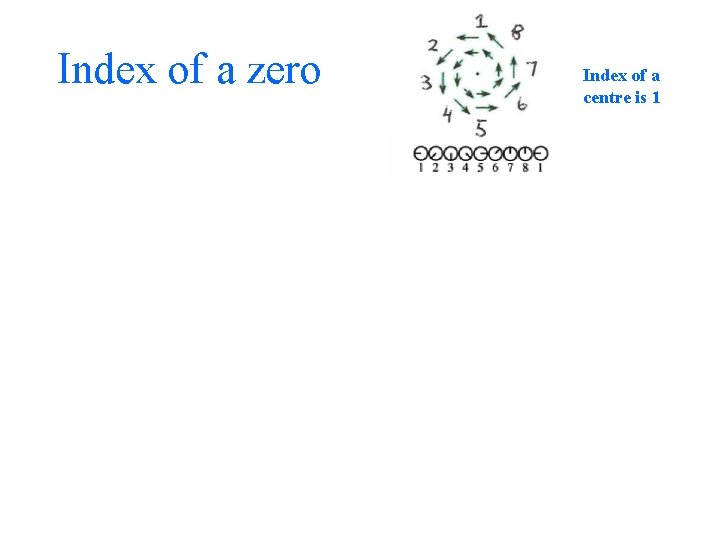Index of a zero Index of a centre is 1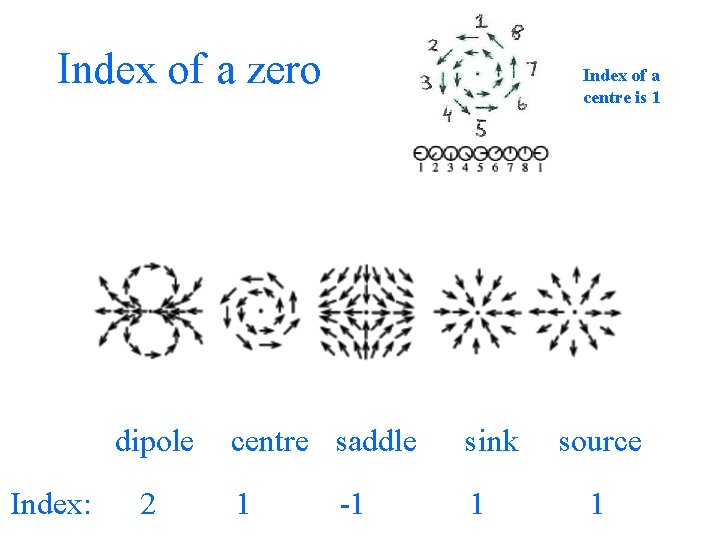Index of a zero dipole Index: 2 Index of a centre is 1 centre saddle sink 1 1 -1 source 1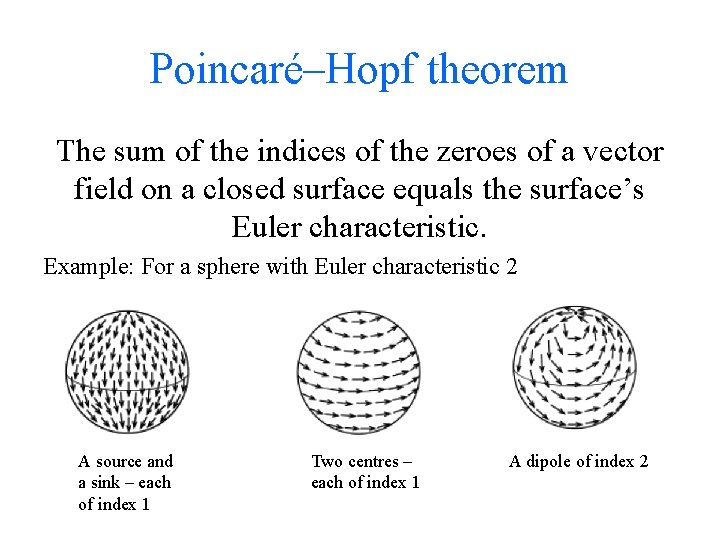Poincaré–Hopf theorem The sum of the indices of the zeroes of a vector field on a closed surface equals the surface’s Euler characteristic. Example: For a sphere with Euler characteristic 2 A source and a sink – each of index 1 Two centres – each of index 1 A dipole of index 2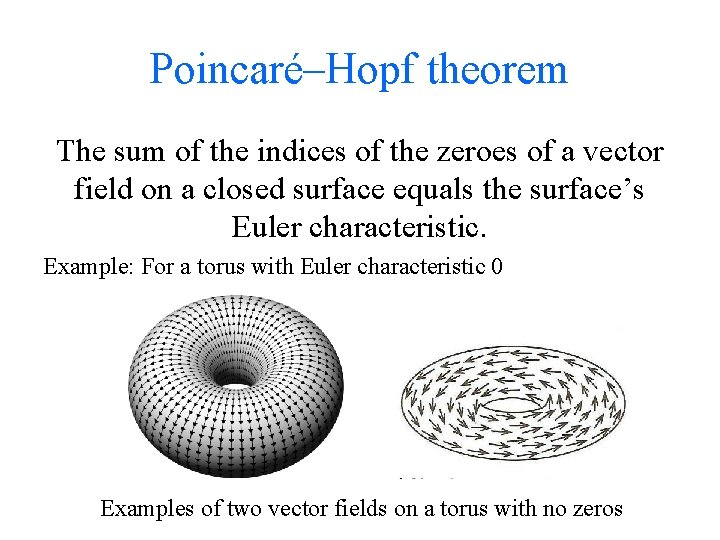Poincaré–Hopf theorem The sum of the indices of the zeroes of a vector field on a closed surface equals the surface’s Euler characteristic. Example: For a torus with Euler characteristic 0 Examples of two vector fields on a torus with no zeros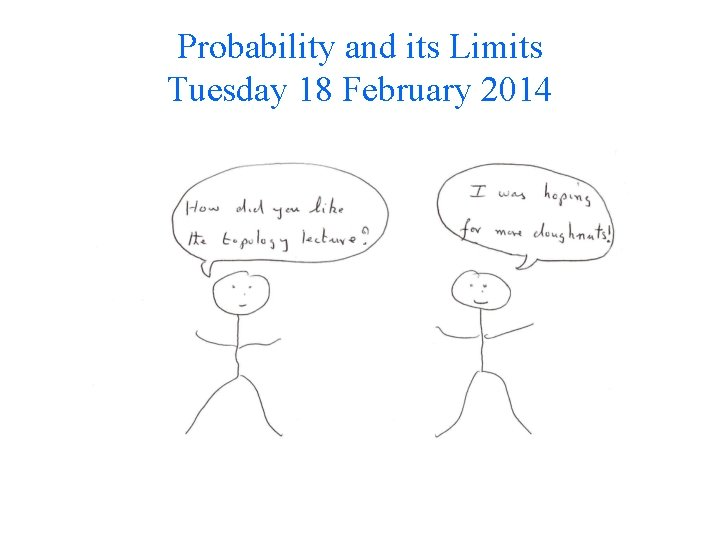Probability and its Limits Tuesday 18 February 2014